The Surface Area Of An Ellipsoid

A. Dieckmann, Universität Bonn, July 2003

This short note shows a way to the formula for the surface area of an ellipsoid. The result is given for example in the formula gallery of the Mathematica Book (Ref. 1), but with a typo. There was no instruction accessible to me, how it is obtained, and I had to try for myself. It proved harder than I first thought, so I think it is worthwhile to put the derivation on the internet.
To make a later integration valid, we have to name the semi-axes of the ellipsoid c > a > b > 0, so that b < c and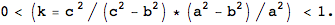The axes may be renamed at the end.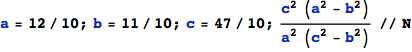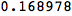Now calculate the area numerically – for reference: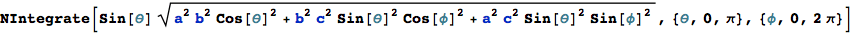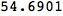Symmetry allows the reduction of the integration domain: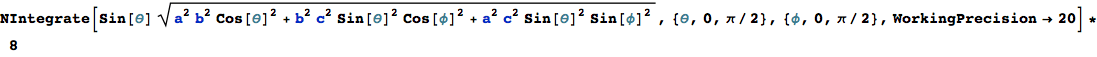Integrate symbolically with respect to θ :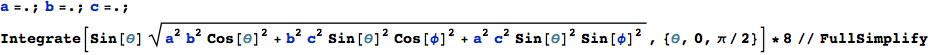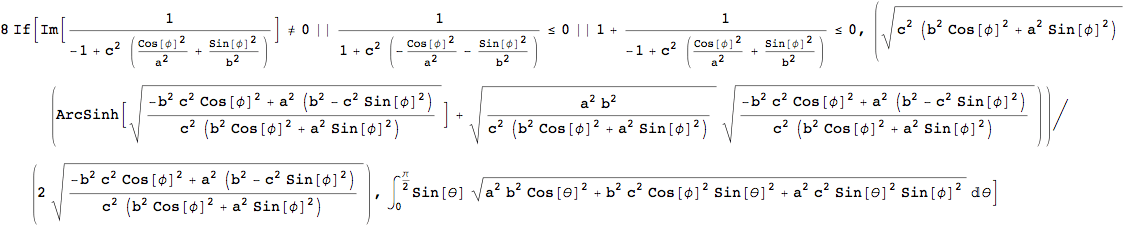After numerical integration with respect to φ the area comes out correctly: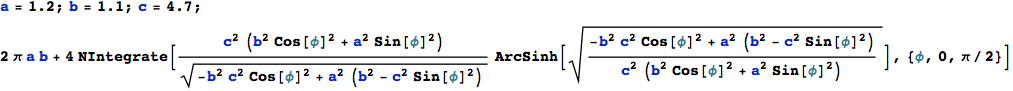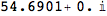Express ArcSinh by ArcTanh: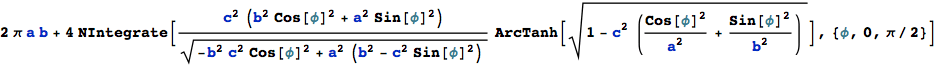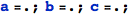And the integral can be reduced to a simpler form by substituting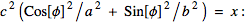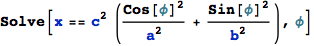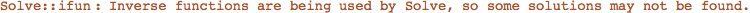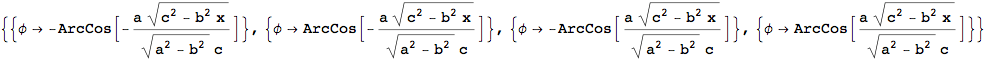The Jacobian dφ/dx is: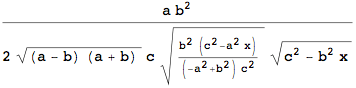Check the new, 'simple' form :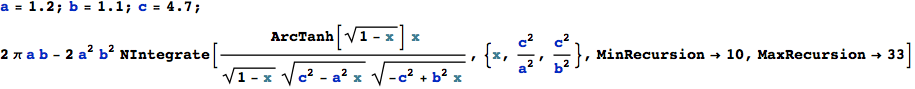Then integrate by parts with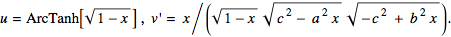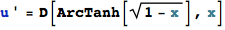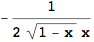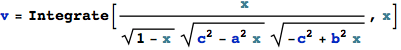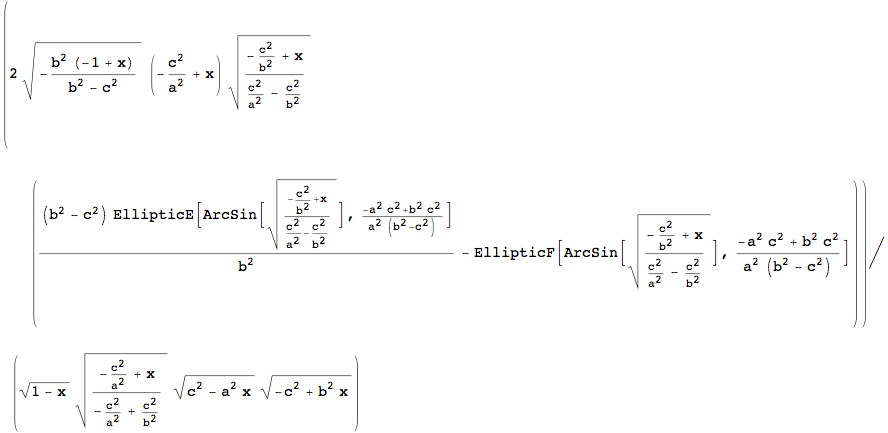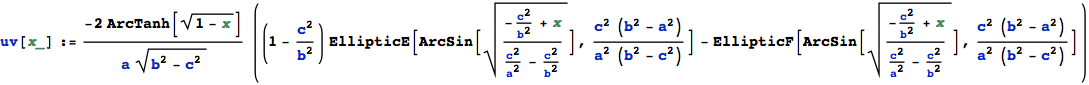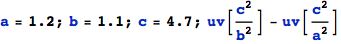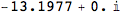uv(upper limit) - uv(lower limit):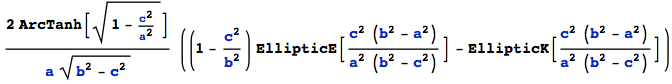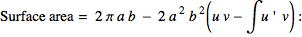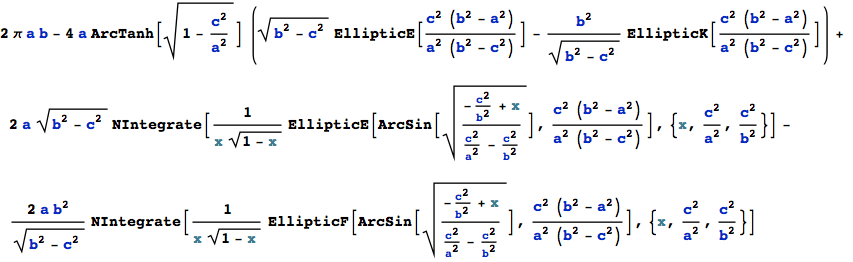There are now two integrals left to attack, but luckily, both have already been done – about 130 years ago. The first is: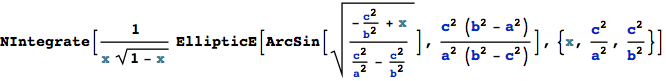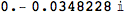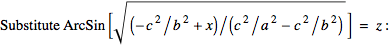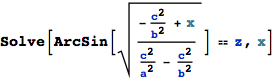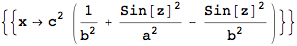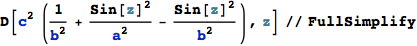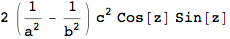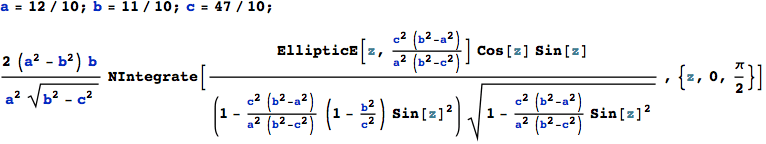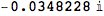If the conditions cited at the beginning
• 0 < ( t = ArcCos[b/c] ) < π/2, or equivalently b < c, and
• 0 < k < 1
are all fulfilled, then this integral has a solution, found with Gradshteyn Ryzhik (6.123) (Ref.2) to be :The second integral is very similar to the first :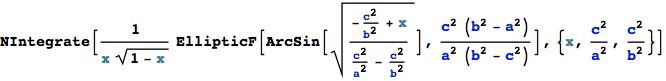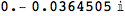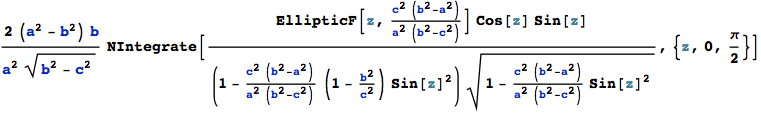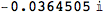This is – with Gradshteyn Ryzhik (6.113-2) – :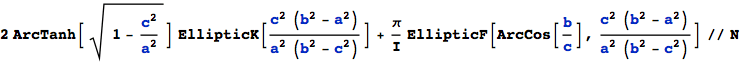Put them into the formula for the surface area above :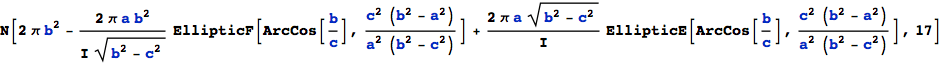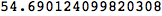This is already the correct expression. Now rename the axes, that a > b > c :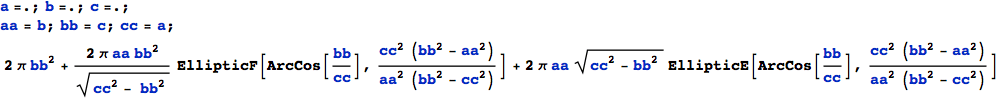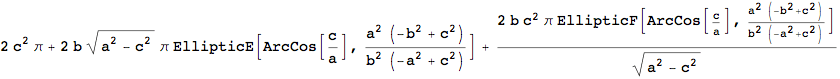The final result is ( incidentally valid for any set of semi-axes – if a ≠ b ≠ c) :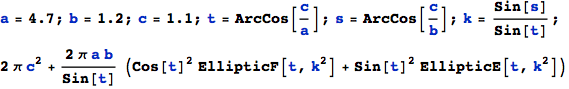Prolate case: b→c ; surface of revolution (radius c)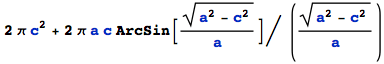Oblate case: b→a ; surface of revolution (radius a)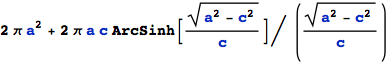c→a ; surface of sphere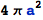As a 'pocket calculator' approximation to the surface area of an ellipsoid, that is better than 1.2% (if a > b ≥ c), we may use: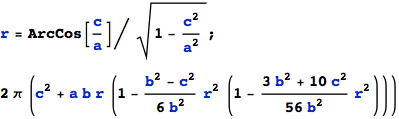This formula is shorter and has a smaller more symmetric error than the one given in a previous version of this note (2003).
Note that the first two terms correspond to the prolate surface of revolution.

References

1. Wolfram: The Mathematica Book, Wolfram Media, Inc., Fourth Edition, 1999
2. Gradshteyn/Ryzhik: Table of Integrals, Series and Products, Academic Press, Second Printing, 1981